### Home > CAAC > Chapter 14 > Lesson 14.3.1.5 > Problem3-69

3-69.
1. Determine which of the following equations are true for all values (always true). For those that are not, decide whether they are true for certain values (sometimes true) or not true for any values (never true). Justify your decisions clearly. Homework Help ✎

1. (x −5)2 = x2 + 25

2. (2x −1)( x + 4) =2x2 + 7x − 4

3.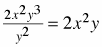4. (3x −2)(2x + 1) = 6x2x − 5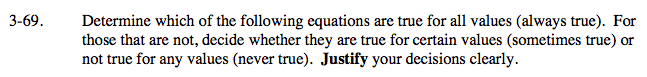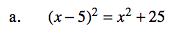Solve the equation. Remember, (x − 5)² = (x − 5)(x − 5).

If the equation has an answer, it is sometimes true.
If the equation has no answer, it is never true.
If the equation is an identity (two ways of writing the same expression), it is always true, unless there is some limiting factor such as a square root or denominator.

Sometimes true. (when x = 0)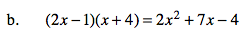Refer to part (a).

Always true.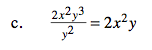Refer to part (a).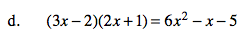Refer to part (a).